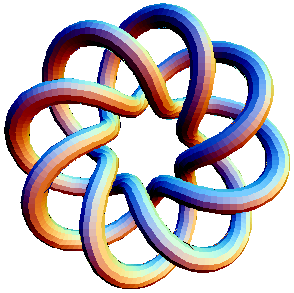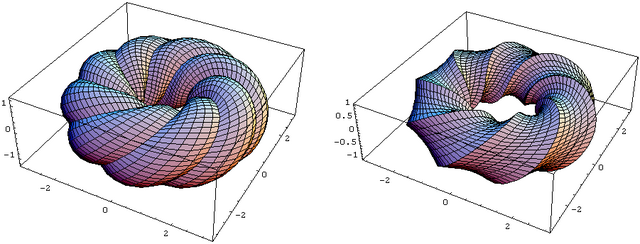# 0708-1300/Class notes for Thursday, January 17

 `In:=` `<< KnotTheory``
 `In:=` `TubePlot[TorusKnot[8, 3]]` `Out=``In:=` ```TC[r1_, t1_,r2_,t2_ ] := { (r1 +r2 Cos[2Pi t2])Cos[2Pi t1], (r1 +r2 Cos[2Pi t2])Sin[2Pi t1], r2 Sin[2Pi t2] };```
 `In:=` ```InflatedTorus[p_, q_, b_] := ParametricPlot3D[ TC[ 2, p t - q s, 1 + b(p^2 + q^2)s(1 - (p^2 + q^2)s), q t + p s ], {t, 0, 1}, {s, 0, 1/(p^2 + q^2)}, PlotPoints -> {6(p^2 + q^2) + 1, 7}, DisplayFunction -> Identity ];```
 `In:=` `GraphicsArray[{{InflatedTorus[3,8,1], InflatedTorus[3,8,-1]}}]` `Out=`